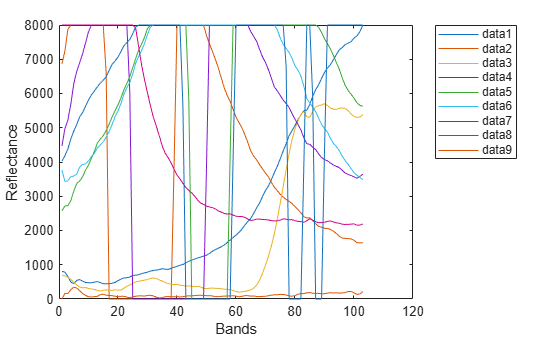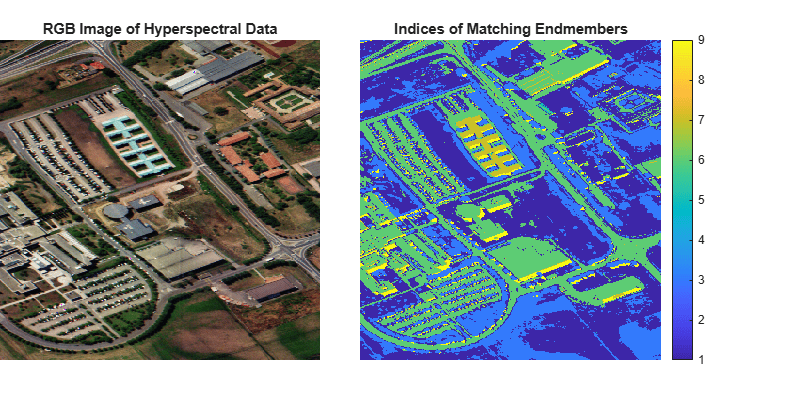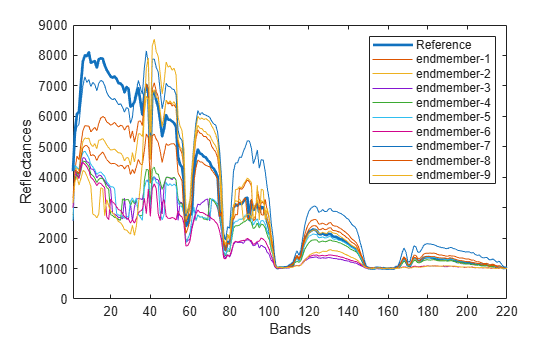# jmsam

Measure spectral similarity using Jeffries Matusita-Spectral Angle Mapper method

Since R2020b

## Syntax

``score = jmsam(inputData,refSpectrum)``
``score = jmsam(testSpectrum,refSpectrum)``

## Description

example

````score = jmsam(inputData,refSpectrum)` measures the spectral similarity between the spectrum of each pixel in the hyperspectral data `inputData` and the specified reference spectrum `refSpectrum` by using Jeffries Matusita-Spectral Angle Mapper (JMSAM) method. Use this syntax to identify different regions or materials in a hyperspectral data cube. For information about the JMSAM method, see More About.```

example

````score = jmsam(testSpectrum,refSpectrum)` measures the spectral similarity between the specified test spectrum `testSpectrum` and reference spectrum `refSpectrum` by using the JMSAM method. Use this syntax to compare the spectral signature of an unknown material against the reference spectrum or to compute spectral variability between two spectral signatures. NoteThis function requires the Image Processing Toolbox™ Hyperspectral Imaging Library. You can install the Image Processing Toolbox Hyperspectral Imaging Library from Add-On Explorer. For more information about installing add-ons, see Get and Manage Add-Ons.The Image Processing Toolbox Hyperspectral Imaging Library requires desktop MATLAB®, as MATLAB Online™ or MATLAB Mobile™ do not support the library. ```

## Examples

collapse all

Read hyperspectral data into the workspace.

`hcube = hypercube('paviaU.dat');`

Extract the first 9 endmember spectral signatures from the data cube by using the NFINDR algorithm.

```numEndmembers = 9; endmembers = nfindr(hcube,numEndmembers);```

Plot the spectral signatures of the extracted endmembers.

```figure plot(endmembers) xlabel('Bands') ylabel('Reflectance') legend('Location','Bestoutside')```Compute the JM-SAM distance between each endmember and the spectrum of each pixel in the data cube.

```score = zeros(size(hcube.DataCube,1),size(hcube.DataCube,2),numEndmembers); for i = 1:numEndmembers score(:,:,i) = jmsam(hcube,endmembers(:,i)); end```

Compute the minimum score value from the distance scores obtained for each pixel spectrum with respect to all the endmembers. The index of each minimum score identifies the endmember spectrum to which a pixel spectrum exhibits maximum similarity. An index value, n, at the spatial location (x, y) in the score matrix indicates that the spectral signature of the pixel at spatial location (x, y) in the data cube best matches the spectral signature of the nth endmember.

`[~,matchingIdx] = min(score,[],3);`

Estimate an RGB image of the input data by using the `colorize` function.

`rgbImg = colorize(hcube,'Method','rgb','ContrastStretching',true);`

Display both the RGB image and the matrix of matched index values.

```figure('Position',[0 0 800 400]) subplot('Position',[0 0.1 0.4 0.8]) imagesc(rgbImg) axis off title('RGB Image of Hyperspectral Data') subplot('Position',[0.45 0.1 0.45 0.8]) imagesc(matchingIdx) axis off title('Indices of Matching Endmembers') colorbar```Read hyperspectral data into the workspace.

`hcube = hypercube('indian_pines.dat');`

Find the first 10 endmembers of the hyperspectral data.

```numEndmembers = 10; endmembers = nfindr(hcube,numEndmembers);```

Consider the first endmember as the reference spectrum and the rest of the endmembers as the test spectra.

```refSpectrum = endmembers(:,1); testSpectra = endmembers(:,2:end);```

Plot the reference spectrum and other endmember spectra.

```figure plot(refSpectrum,'LineWidth',2) hold on plot(testSpectra) hold off label = cell(1,numEndmembers-1); label{1} = 'Reference'; for itr = 1:numEndmembers-1 label{itr+1} = ['endmember-' num2str(itr)]; end xlabel('Bands') ylabel('Reflectances') legend(label) xlim([1 size(hcube.DataCube,3)]);```Compute the JM-SAM score between the reference and test spectra.

```score = zeros(1,numEndmembers-1); for itr = 1:numEndmembers-1 score(itr) = jmsam(testSpectra(:,itr),refSpectrum); end```

Find the test spectrum that exhibit maximum similarity (minimum distance) to the reference spectrum. Then find the test spectrum that exhibit minimum similarity (maximum distance) to the reference spectrum.

```[minval,minidx] = min(score); maxMatch = testSpectra(:,minidx); [maxval,maxidx] = max(score); minMatch = testSpectra(:,maxidx);```

Plot the reference spectrum, the maximum similarity, and the minimum similarity test spectrum. The test spectrum with the minimum score value indicates highest similarity to the reference endmember. On the other hand, the test spectrum with the maximum score value has the highest spectral variability and characterises the spectral behaviour of two different materials.

```figure plot(refSpectrum,'LineWidth',2) hold on plot(maxMatch,'k') plot(minMatch,'r') xlabel('Band Number') ylabel('Data Values') xlim([1 size(hcube.DataCube,3)]); legend('Reference spectrum','Maximum match test spectrum','Minimum match test spectrum') title('Similarity Between Spectra') text(5,1000,['Max score: ' num2str(maxval)],'Color','r') text(140,3000,['Min score: ' num2str(minval)],'Color','k')```## Input Arguments

collapse all

Input hyperspectral data, specified as a `hypercube` object or a 3-D numeric array containing the data cube. If the input is a `hypercube` object, the data is read from the `DataCube` property of the object.

Test spectrum, specified as a C-element vector. The test spectrum is the spectral signature of an unknown region or material.

Data Types: `single` | `double` | `int8` | `int16` | `int32` | `int64` | `uint8` | `uint16` | `uint32` | `uint64`

Reference spectrum, specified as a C-element vector. The reference spectrum is the spectral signature of a known region or material. The function matches the test spectrum against these values.

Data Types: `single` | `double` | `int8` | `int16` | `int32` | `int64` | `uint8` | `uint16` | `uint32` | `uint64`

## Output Arguments

collapse all

JMSAM score, returned as a scalar or matrix. The output is a

• scalar — If you specify the `testSpectrum` input argument. The function matches the test spectral signature against the reference spectral signature and returns a scalar value. Both the test and the reference spectra must be vectors of same length.

• matrix — If you specify the `inputData` input argument. The function matches the spectral signature of each pixel in the data cube against the reference spectral signature and returns a matrix. If the data cube is of size M-by-N-by-C and the reference spectra is a vector of length C, the output matrix is of size M-by-N.

A smaller JMSAM score indicates a strong match between the test signature and the reference signature.

Data Types: `single` | `double`

## Limitations

This function does not support `parfor` loops, as its performance is already optimized. (since R2023a)

collapse all

### Jeffries Matusita-Spectral Angle Mapper (JMSAM)

The JMSAM method computes spectral similarity based on the Jeffries Matusita (JM) and SAM distances between two spectra. Let r and t be the reference and test spectra respectively.

First, compute the JM distance,

`$J{M}_{\text{distance}}=2\left(1-{e}^{-B}\right)$`

where B is the Bhattacharyya distance,

`$B=\frac{1}{8}{\left({\mu }_{t}-{\mu }_{r}\right)}^{T}{\left[\frac{{\sigma }_{t}+{\sigma }_{r}}{2}\right]}^{-1}\left({\mu }_{t}-{\mu }_{r}\right)\text{\hspace{0.17em}}+\text{\hspace{0.17em}}\frac{1}{2}\mathrm{ln}\left[\frac{|\frac{{\sigma }_{t}+{\sigma }_{r}}{2}|}{\sqrt{|{\sigma }_{t}||{\sigma }_{r}|}}\right]$`

μr and μt are the mean values of the reference and test spectra respectively. σr and σt are the covariance values of the reference and test spectra respectively.

Then, compute the SAM value α by using the test spectra t and a reference spectra r of length C,

`$\alpha =\text{\hspace{0.17em}}{\mathrm{cos}}^{-1}\left(\frac{\sum _{i=1}^{C}{t}_{i}{r}_{i}}{\sqrt{\sum _{i=1}^{C}{t}_{i}^{2}}\sqrt{\sum _{i=1}^{C}{r}_{i}^{2}}}\right).$`

Finally, compute the JMSAM score as:

`$JMSAM\text{\hspace{0.17em}}=\text{\hspace{0.17em}}\text{\hspace{0.17em}}J{M}_{distance}×\mathrm{tan}\left(\alpha \right)\text{\hspace{0.17em}}$`

 Padma, S., and S. Sanjeevi. “Jeffries Matusita Based Mixed-Measure for Improved Spectral Matching in Hyperspectral Image Analysis.” International Journal of Applied Earth Observation and Geoinformation 32 (October 2014): 138–51. https://doi.org/10.1016/j.jag.2014.04.001.